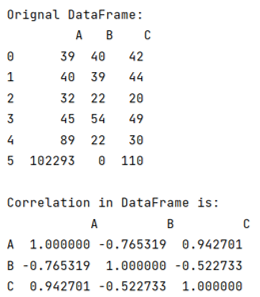# How to use corr() to get the correlation between two columns?

By using the corr() method, learn how to get the correlation between two columns? By Pranit Sharma Last updated : September 22, 2023

Pandas is a special tool that allows us to perform complex manipulations of data effectively and efficiently. Inside pandas, we mostly deal with a dataset in the form of DataFrame. DataFrames are 2-dimensional data structures in pandas. DataFrames consist of rows, columns, and the data.

## Use of corr() to get the correlation between two columns

There is always some kind of similarity/difference between all the values of all the columns in pandas DataFrame. This similarity or difference is known as the correlation of values in a DataFrame. To find the correlation in pandas, we use pandas.DataFrame.corr() method in pandas.

The DataFrame.corr() method is used to find the pair-wise correlation (similarities / differences) of the column values. The important point is if there is any null value present in any column, DataFrame.corr() automatically excludes it and also the non-numeric data is ignored.

Syntax:

```DataFrame.corr(
method='pearson',
min_periods=1
)
```
Note

To work with pandas, we need to import pandas package first, below is the syntax:

```import pandas as pd
```

Let us understand with the help of an example,

## Python program to get the correlation between two columns

```# Importing pandas package
import pandas as pd

# Importing seaborn package
import seaborn as sn

# Import matplotlib package
import matplotlib.pyplot as plt

# Create a DataFrame
df = pd.DataFrame({
'A':[39,40,32,45,89,102293],
'B':[40,39,22,54,22,0],
'C':[42,44,20,49,30,110]
})

# Display original DataFrame
print("Original DataFrame:\n",df,"\n")

# Finding correlation
result = df.corr(method ='pearson')

# Display result
print("Correlation in DataFrame is:\n",result,"\n")
```

### Output

The output of the above program is: